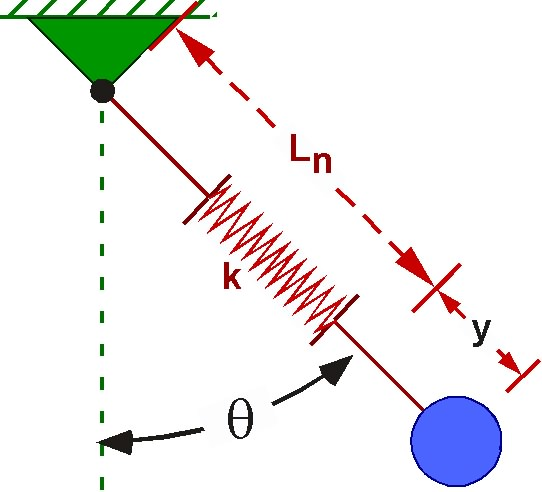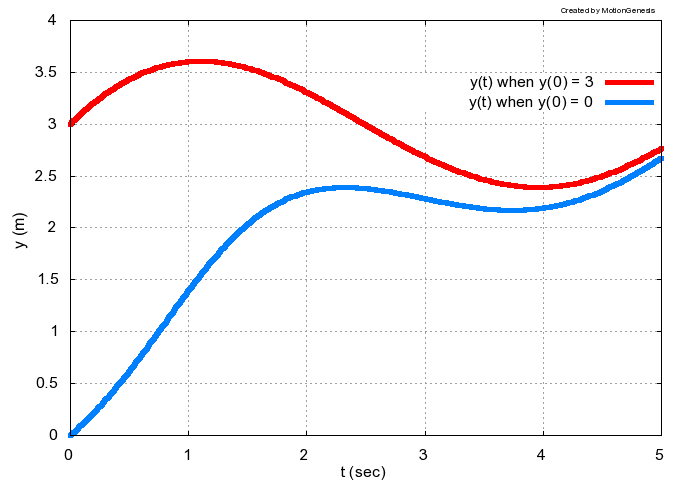Solve ODEs   (Ordinary Differential Equations)

 Solve a nonlinear 2nd-order ODE To solve the ODE shown right with initial values of x = 1 m   and   x' = 0.2 m/s,   for   t = 20 seconds, and to Output   and   Plot   various quantities (with units),   type: ```Variable x'' = cos(2*t) + sin(x) Input x = 1 m, x' = 0.2 m/s, tFinal = 20 sec OutputPlot t sec, x m, x' m/s, x'' km/s^2 ODE() SecondOrderODE ``` Note: The prime symbol ' denotes differentiation with respect to t. Commands may be entered from a text file, e.g.,   SecondOrderODE.txt. Change   SecondOrderODE   to   SecondOrderODE.m   to auto-generate a MATLABÆ .m file.
x''   =   cos(2 * t)   +   sin(x)Plot with   MotionGenesisô or MATLABÆ. The output file   SecondOrderODE.1   stores x(t), etc.

 Solve coupled nonlinear 1st-order ODEs
 Shown below are ODEs for the angular velocity measures   wx, wy, wz for the spinning-book (shown right) along with the commands to Input  initial values and numerical integration parameters and to Output  and   Plot   wx, wy, wz   via the  ODE  command. ```Constant Ixx = 1 kg*m^2, Iyy = 2 kg*m^2, Izz = 3 kg*m^2 Variable wx', wy', wz' wx' = (Iyy-Izz) * wy * wz / Ixx wy' = (Izz-Ixx) * wx * wz / Iyy wz' = (Ixx-Iyy) * wx * wy / Izz Input wx = 0.2 rad/sec, wy = 7.0 rad/sec, wz = 0.2 rad/sec Input tFinal = 4 sec, tStep = 0.02 sec OutputPlot t sec, wx rad/sec, wy rad/sec, wz rad/sec ODE() CoupledODESFirstOrder ``` Note: The prime symbol ' denotes differentiation with respect to t. Note: Commands may be entered from a text file, e.g.,   CoupledODEs.txt. Note: Change   CoupledODEs   to   CoupledODEs.m   to auto-generate a MATLABÆ .m file. Note: MotionGenesisô forms and solves these   dynamics equations here. Note: Video and information about 3D spin-stability and Dzhanibekov effect here.Plot with   MotionGenesisô or MATLABÆ. The output file   CoupledODEs.1   stores t, wx, wy, wz.

 Solve coupled 2nd-order ODEs Shown below are the ODEs for   y   and   theta   that govern motions of the swinging-spring (shown right) along with the MotionGenesisô commands to Input  initial values and numerical integration parameters and to Output  and   Plot   theta vs. t   via the  ODE  command. ```Constant m = 1 kg, g = 9.8 m/s^2, k = 100 N/m, Ln = 0.3 m Variable y'', theta'' y'' = g * cos(theta) + (Ln+y) * theta'^2 - k*y/m theta'' = -(g*sin(theta) + 2*theta'*y') / (Ln+y ) Input y = 0.2 m, y' = 0, theta = 1 deg, theta' = 0 Input tFinal = 15 sec, tStep = 0.02 sec OutputPlot t sec, theta degrees ODE() SwingingSpring ``` Note: Commands may be entered from a text file, e.g.,   SwingingSpring.txt. Note: Change   SwingingSpring   to   SwingingSpring.m   to auto-generate a MATLABÆ .m file. Note: MotionGenesisô forms these   dynamics equations here.Plot with   MotionGenesisô or MATLABÆ. The output file   SwingingSpring.1   stores the solution.
 MotionGenesisô generates fast compact symbolically-optimized code to solve ODEs Code Command and code file Comments MATLABÆ ODE() SwingingSpring.m Modify input values in   SwingingSpring.m Note: Interpreted .m codes may be slower than compiled codes. C Fortran ODE() SwingingSpring.c ODE() SwingingSpring.f Compile and link source code. Modify input values in   SwingingSpring.in Note: Compiled codes can optimize for its host processor.
 Depending on your   license,   these   valuable versatile   codes are independent of MotionGenesisô.

 Solve an ODE with an on/off if-like statement MotionGenesisô commands to solve the ODE:    if( t < 𝛑 )   y' = sin(t)   else y' = 0
```isTLessThanPi = isPositive(pi-t)      % true   if t < pi, otherwise 0 (false).
sintOrZero = sin(t) * isTLessThanPi   % sin(t) if t < pi, otherwise 0 (false).
Variable y' = sintOrZero
Input  tFinal = 4,  y = 0             % Arbitrary input values.
Output  t,  y,  y'
ODE()  simulateWithIfStatement
% Plot simulateWithIfStatement.1[1, 2, 3]
```

Solve ODE by auto-generating MATLABÆ or C code and pass an argument to that program.
 Shown below are the MotionGenesisô commands to solve the ODEs for x and y that govern projectile motion of a baseball. Initial values are specified in the input command and the OutputPlot command plots   y vs. x   after the  ODE  command is run. These commands auto-generate the MATLABÆ file   MGProjectileMotion.m To run from the MATLABÆ prompt, type:   MGProjectileMotion( 0.05 ) ```Constant m = 145 grams, g = 9.8 m/s^2, b Variable x'' = -b*x'/m Variable y'' = -g - b*y'/m %------------------------------------------- Input x = 0 m, x' = 44.7*cosDegrees(30) m/s Input y = 0 m, y' = 44.7*sinDegrees(30) m/s Input tFinal = 3.8 s OutputPlot x meter, y meter ODE() MGProjectileMotion.m( b ) ```Plot with   MotionGenesisô or MATLABÆ.
Commands may be entered from a   text file.
MotionGenesisô forms these   dynamics equations here.
Note:  To pass  b,  to the C-program   MGProjectileMotion.c,  type:
ODE() MGProjectileMotion.c( b )
After compiling, run from the operating system prompt by typing:
MGProjectileMotion   0.05

 Solve an ODE with various initial values
```Variable  y' = cos(t) + sin(y)  % Arbitrary ODE.
Output  t sec,  y m,  y' m/s    % List output quantities  (for subsequent ODE command).
Input   y = 3 m                 % Set initial value for y (for subsequent ODE command).
%--------------------------------------------------------------------
%   Set numerical integration parameters and solve the ODE with initial value y = 3.
Input  tFinal = 5 sec,  tStep = 0.02 sec,  absError = 1.0E-07
ODE()  ODEWithInitialValueA     % Solve the ODE and output results.
%--------------------------------------------------------------------
Input  y := 0 m                 % Modify the initial value for y.
ODE()  ODEWithInitialValueB     % Solve the ODE and output results.
%--------------------------------------------------------------------
%   Optional: Plot results
Plot ODEWithInitialValueA.1 [1,2]  ODEWithInitialValueB.1 [1,2]
```Commands may be entered from a text file, e.g., MGSolveODEWithVariousInitialValues.txt.